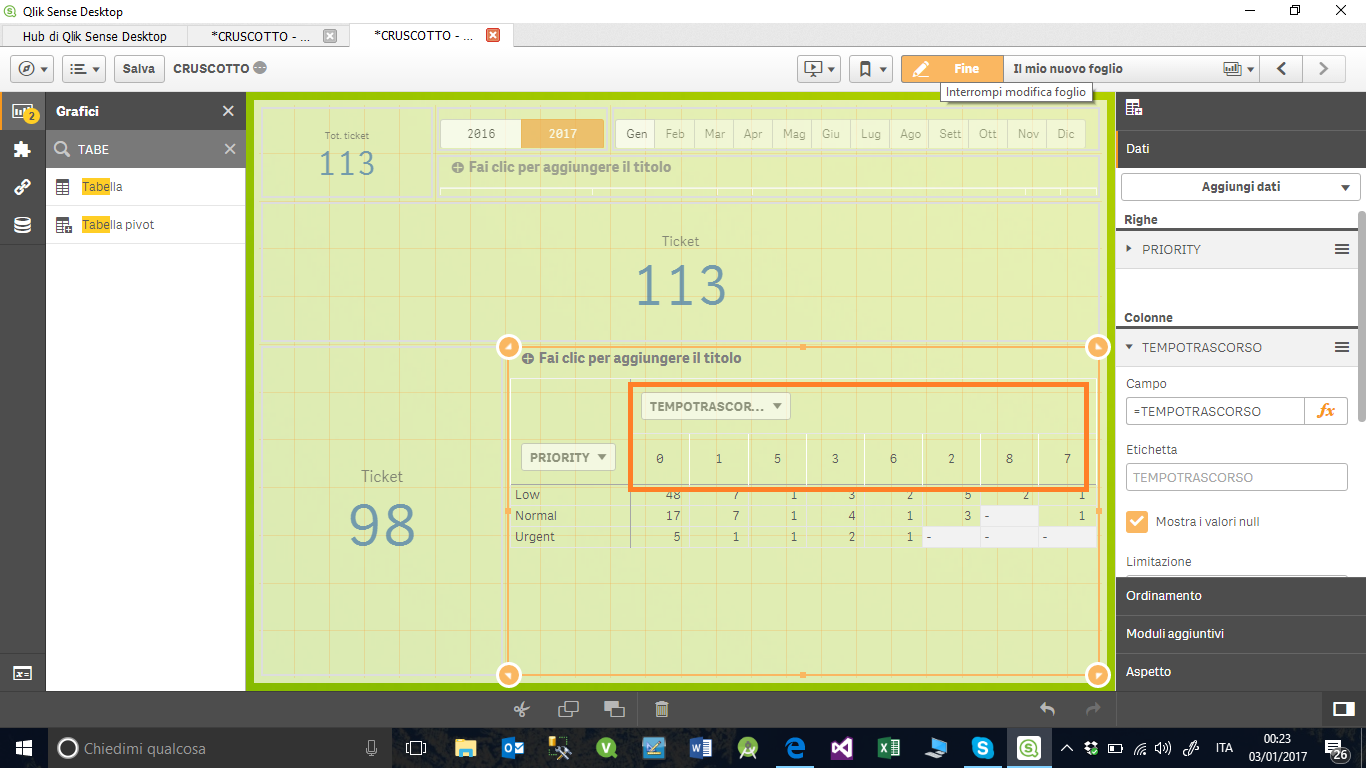# New to Qlik Sense

Discussion board where members can get started with Qlik Sense.

Announcements
Qlik® Product Spotlight: Discover what’s possible. Get more from our products.
See for yourself. Register today.
Not applicable

## LOAD ... CASE

Hi Community,

only a question.

May I use "case statement" in load script?

I think not but could you confirm this

DELTA,

TEMPOTRASCORSO,

CASE

WHEN TEMPOTRASCORSO >= 0 AND <=4

THEN

'0>= Risolto <=4'

WHEN (TEMPOTRASCORSO) > 5 AND (TEMPOTRASCORSO) <= 8

THEN

'>5H Risolto <=8'

ELSE

'Risolto > 8h'

END AS GRIGLIATEMPO;

6 Replies
Not applicable

## Re: LOAD ... CASE

May be using an if statement

DELTA,

TEMPOTRASCORSO,

If(TEMPOTRASCORSO >= 0 and TEMPOTRASCORSO <= 4, '0>= Risolto <=4',

If(TEMPOTRASCORSO > 5 and TEMPOTRASCORSO <= 8, '>5H Risolto <=8', 'Risolto > 8h')) as GRIGLIATEMPO;

Not applicable

## Re: LOAD ... CASE

Hi,

thanks for your reply but the result is not as hoped

My data is:

Status ticket      Closed time

open     1                    8

open     2                    3

open     3                    14

open     4                     24

I need to create a table

raw: status

column: Closed Time (>= 0 and <=4)

column: Closed Time (>=5 and <= 8)

column: Closed Time (>=9 and <= 24)

etc

measure : count (ticket)

So that

---------N°  ticket ---------------------------

status                  0 and 4        | 5 and 8      | 9 and 24|

open                       1                     1                   2

Instead my data is not groupedNot applicable

## Re: LOAD ... CASE

Hi

Please try below modified script.

DELTA,

TEMPOTRASCORSO,

If(TEMPOTRASCORSO >= 0 and TEMPOTRASCORSO <= 4, '0 and 4',

If(TEMPOTRASCORSO >= 5 and TEMPOTRASCORSO <= 8, '5 and 8', '9 and 24)) as GRIGLIATEMPO;

You have to use 'GRIGLIATEMPO' in column group.

Not applicable

## Re: LOAD ... CASE

Hi,

Try like this

Status,

ticket.

[Closed time],

If([Closed time] >= 0 AND [Closed time] <= 4, '0 and 4',

If([Closed time] >= 5 AND [Closed time] <= 8, '5 and 8',

If([Closed time] >= 9 AND [Closed time] <= 24, '9 and 24', 'N/A'))) AS TimeBucket

FROM DataSource;

Not applicable

## Re: LOAD ... CASE

Hi

excuse me last night it was late and I had not realised the error.

Last question:

If I run the script It indicates the TEMPOTRASCORSO field is NOT FOUND this because it is a computed field with this formula

num (SubField ((DTDELTA), '' .1)) * 24 + num (SubField (SubField (.2 (DTDELTA), ' '), ': ' .1))     AS "TEMPOTRASCORSO"

Exist a syntax in order to have this field in  if you suggested?

Not applicable

## Re: LOAD ... CASE

Move it to the preceding load

DELTA,

TEMPOTRASCORSO,

If(TEMPOTRASCORSO >= 0 and TEMPOTRASCORSO <= 4, '0>= Risolto <=4',

If(TEMPOTRASCORSO > 5 and TEMPOTRASCORSO <= 8, '>5H Risolto <=8', 'Risolto > 8h')) as GRIGLIATEMPO;